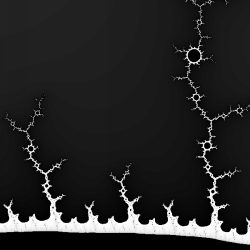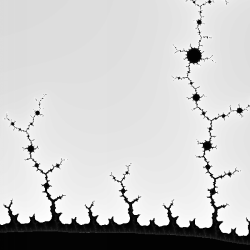Theory.org » intro » 2ndary » Secondary Fractals / Fractal Secondaries

 These two fractals are identical except for the use of slightly different palettes. They represent a Julia set for a c-value taken inside a secondary Mandelbrot set. A secondary Mandelbrot is a mandelbrot that occurs inside a dendrite coming off the main Mandelbrot. A ternary Mandelbrot would be inside the dendrite coming out of the secondary Mandelbrot; etc. Each of the inner circles --- seen inside the dendritic branches below --- could be thought of as a secondary Julia. The vacuole at the bottom of the images is the primary Julia vacuole. Is there a relationship between a secondary (ternary, etc.) Julia and a structure in the Mandelbrot set (say, ternary (quadrary, etc.) ) Mandelbrots? Use XaoS to find out.-0.000060 < x < +0.000050 :: p = -0.15652
+0.083936 < y < +0.084046 :: q = -1.03225

 f(z) = z2 + c z = x + i*y c = p + i*q

 c-value - A c-value is a function parameter. For example, let's take the function: x2 - c. We will define x to be initially zero and we will start out with our c-value, our paramater, to be one. Lets, iterate this function! ```x0 = 02 - 1 = 0 - 1 = -1 x1 = -12 - 1 = 1 - 1 = 0 x2 = 02 - 1 = 0 - 1 = -1 x3 = -12 - 1 = 1 - 1 = 0 x4 = 02 - 1 = 0 - 1 = -1 ``` Apparently this paramater, or c-value, gives us an oscillator. What about c=3? ```x0 = 02 - 3 = 0 - 3 = -3 x1 = -32 - 3 = 9 - 3 = 6 x2 = 62 - 3 = 36 - 3 = 33 x3 = 332 - 3 = 1089 - 3 = 1086 x4 = 10862 - 3 = 1179396 - 3 = 1179393 ``` Looks like this is going to head out to infinity. Thus, different c-values can give different behaviors. What would happen for c=2?

If you have any questions, don't hesitate to email me!
Jeremy Avnet : : brainsik(at)theory.org
Revised: 22nd March 1999
Created: 7th August 1998